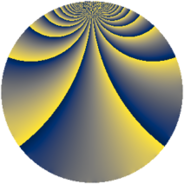# Properties

 Label 1210.2.gLevel $1210$ Weight $2$ Character orbit 1210.g Rep. character $\chi_{1210}(81,\cdot)$ Character field $\Q(\zeta_{5})$ Dimension $144$ Sturm bound $396$

# Related objects

## Defining parameters

 Level: $$N$$ $$=$$ $$1210 = 2 \cdot 5 \cdot 11^{2}$$ Weight: $$k$$ $$=$$ $$2$$ Character orbit: $$[\chi]$$ $$=$$ 1210.g (of order $$5$$ and degree $$4$$) Character conductor: $$\operatorname{cond}(\chi)$$ $$=$$ $$11$$ Character field: $$\Q(\zeta_{5})$$ Sturm bound: $$396$$

## Dimensions

The following table gives the dimensions of various subspaces of $$M_{2}(1210, [\chi])$$.

Total New Old
Modular forms 888 144 744
Cusp forms 696 144 552
Eisenstein series 192 0 192

## Trace form

 $$144q - 2q^{2} - 4q^{3} - 36q^{4} + 6q^{6} - 2q^{8} - 28q^{9} + O(q^{10})$$ $$144q - 2q^{2} - 4q^{3} - 36q^{4} + 6q^{6} - 2q^{8} - 28q^{9} + 8q^{10} - 4q^{12} + 8q^{13} - 2q^{14} + 12q^{15} - 36q^{16} - 8q^{17} - 16q^{18} + 14q^{19} - 48q^{21} + 6q^{24} - 36q^{25} + 12q^{26} + 26q^{27} + 40q^{29} - 4q^{30} + 8q^{32} + 44q^{34} - 8q^{35} - 38q^{36} + 8q^{37} - 20q^{38} + 32q^{39} - 2q^{40} + 30q^{41} + 8q^{42} - 12q^{43} + 8q^{46} - 36q^{47} - 4q^{48} - 86q^{49} - 2q^{50} - 10q^{51} - 12q^{52} - 8q^{53} - 16q^{54} - 12q^{56} - 70q^{57} - 40q^{58} - 62q^{59} - 8q^{60} + 24q^{61} - 4q^{62} + 44q^{63} - 36q^{64} + 20q^{65} + 44q^{67} - 8q^{68} + 12q^{69} + 8q^{71} + 14q^{72} + 24q^{73} + 4q^{74} + 6q^{75} - 16q^{76} + 96q^{78} - 58q^{81} + 10q^{82} + 34q^{83} + 12q^{84} - 28q^{85} - 42q^{86} + 48q^{87} - 26q^{90} + 94q^{91} + 20q^{92} + 144q^{93} + 26q^{94} - 4q^{96} + 142q^{97} + 8q^{98} + O(q^{100})$$

## Decomposition of $$S_{2}^{\mathrm{new}}(1210, [\chi])$$ into newform subspaces

The newforms in this space have not yet been added to the LMFDB.

## Decomposition of $$S_{2}^{\mathrm{old}}(1210, [\chi])$$ into lower level spaces

$$S_{2}^{\mathrm{old}}(1210, [\chi]) \cong$$ $$S_{2}^{\mathrm{new}}(22, [\chi])$$$$^{\oplus 4}$$$$\oplus$$$$S_{2}^{\mathrm{new}}(55, [\chi])$$$$^{\oplus 4}$$$$\oplus$$$$S_{2}^{\mathrm{new}}(110, [\chi])$$$$^{\oplus 2}$$$$\oplus$$$$S_{2}^{\mathrm{new}}(121, [\chi])$$$$^{\oplus 4}$$$$\oplus$$$$S_{2}^{\mathrm{new}}(242, [\chi])$$$$^{\oplus 2}$$$$\oplus$$$$S_{2}^{\mathrm{new}}(605, [\chi])$$$$^{\oplus 2}$$